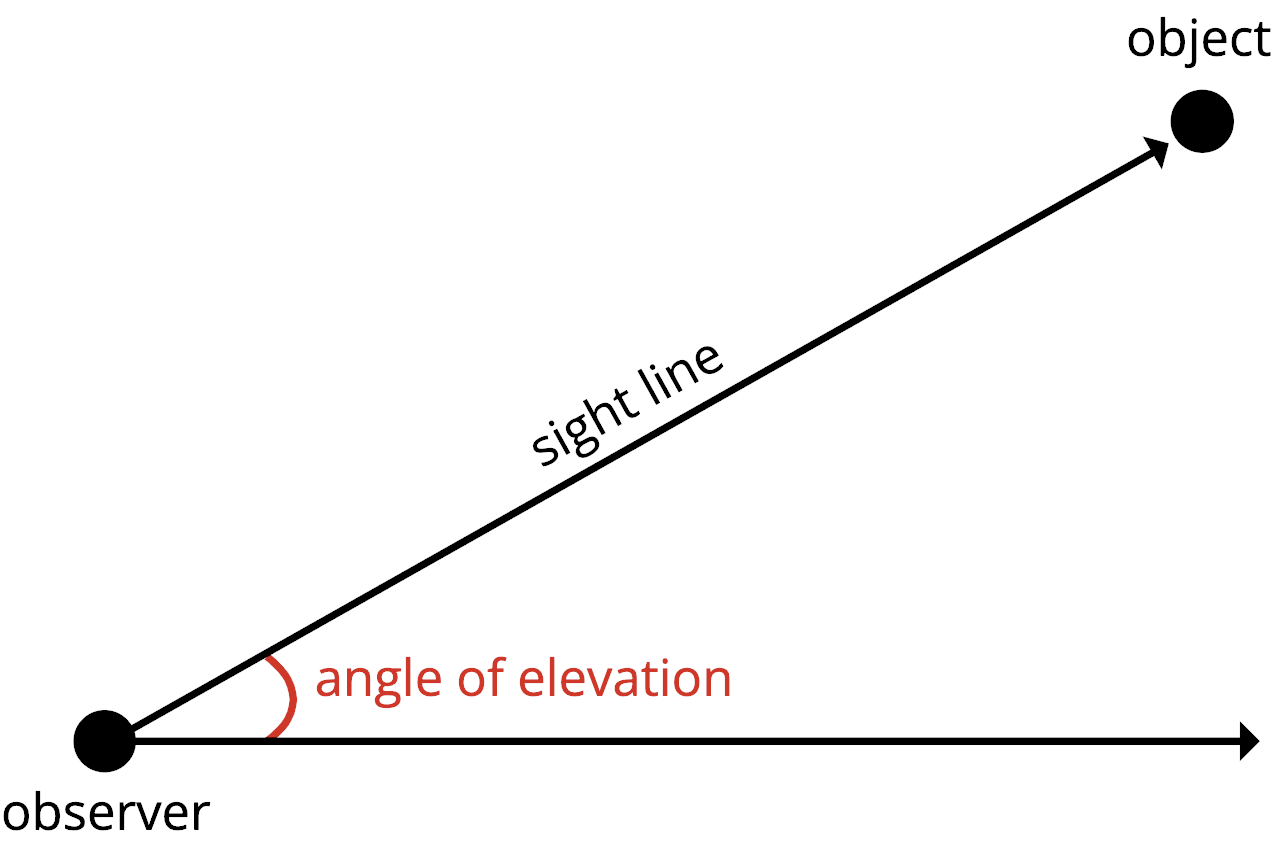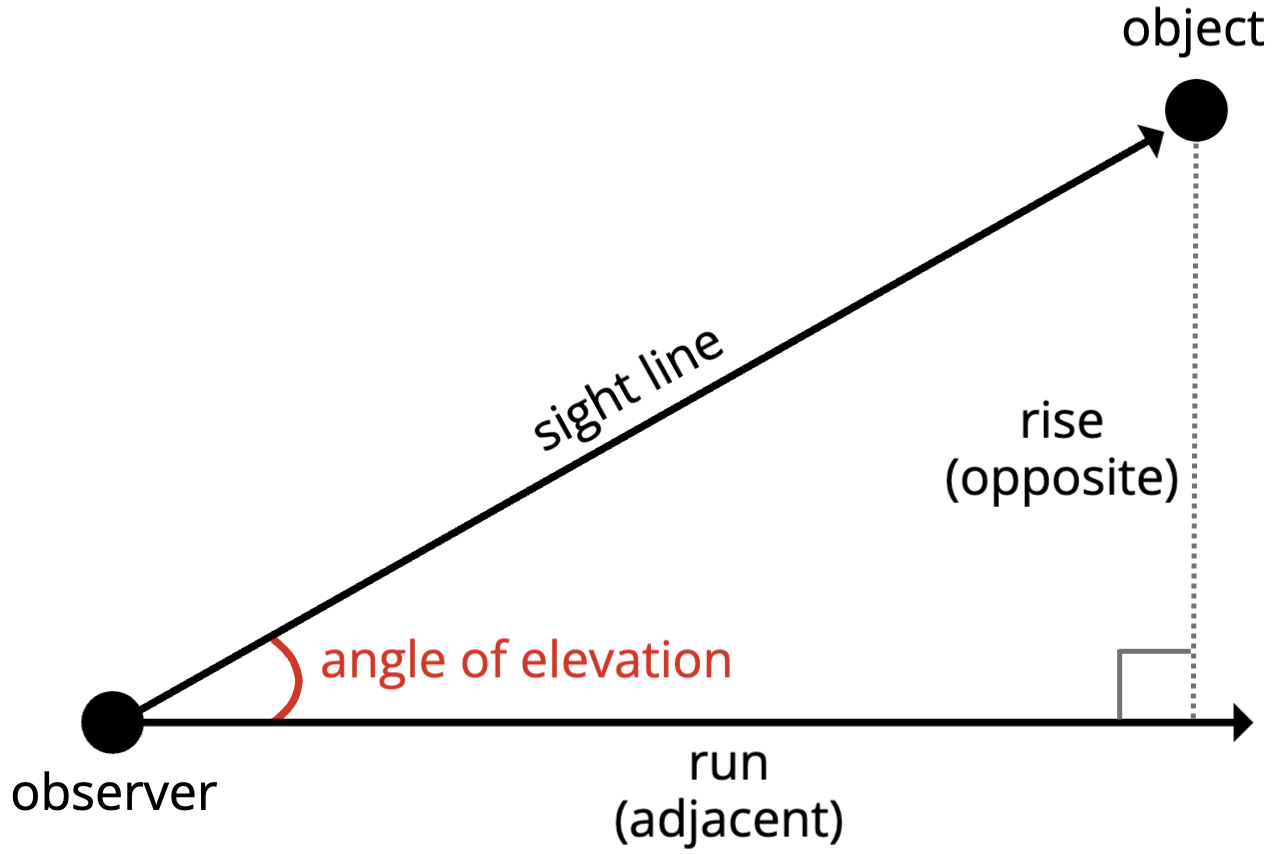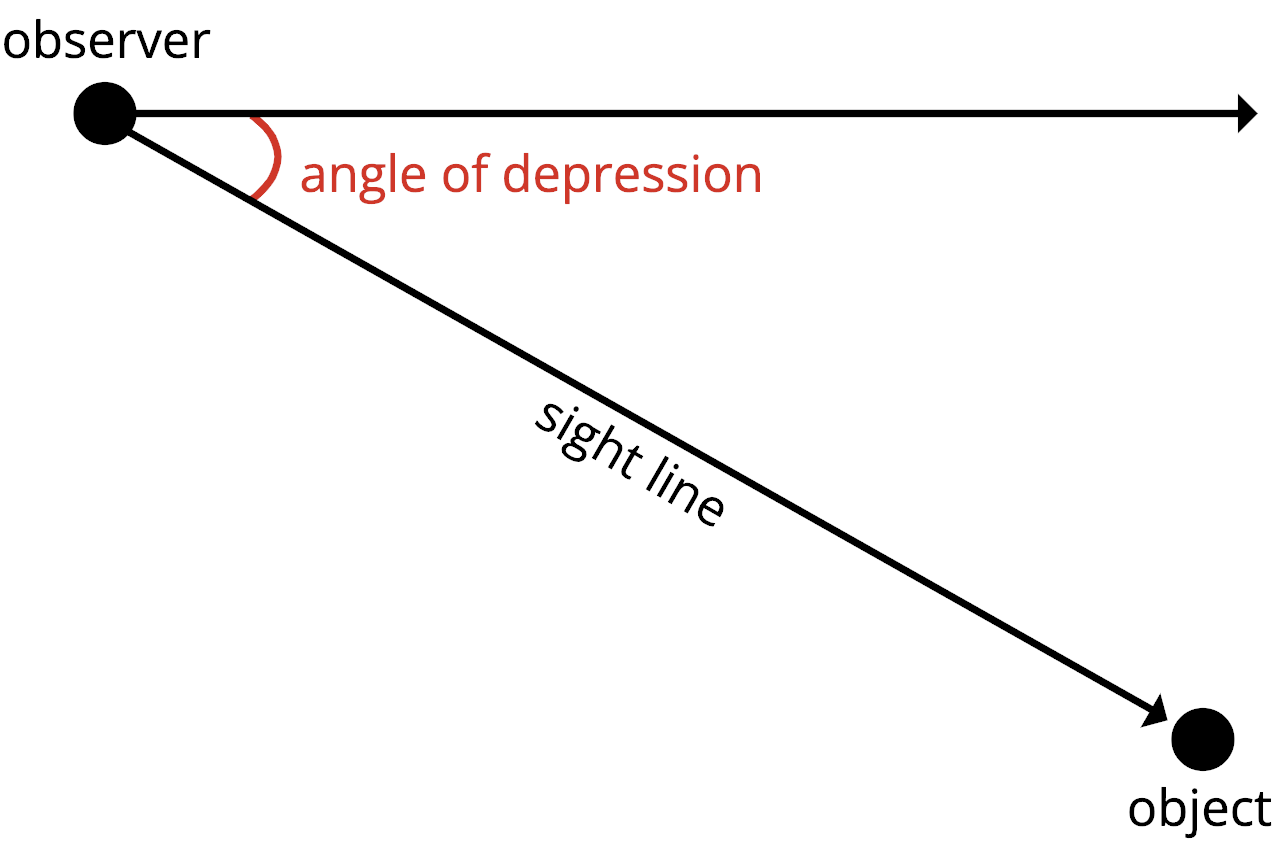# Angle of Elevation Calculator

Calculate the angle of elevation and angle of depression by entering the vertical height (rise) and horizontal distance (run) below.

vertical increase
horizontal distance

## Angle of Elevation:

Learn how we calculated this below

## How to Calculate the Angle of Elevation

Angle of elevation is the positive, or upwards, angle of a line of sight from an observer to an object. For instance, if you were standing outside looking up at the top of a tree, the angle of elevation is the angle your head would need to tilt in order to look at the top of the tree.You can calculate the angle of elevation using trigonometry. Considering that the line of sight and the horizontal baseline form a right triangle, you can use a simple trig function to calculate the angle of elevation.

### Angle of Elevation Formula

The formula to calculate the angle of elevation is:

The tangent of the angle θ is equal to the length of the opposite side divided by the length of the adjacent side. If you substitute the vertical height (opposite) and horizontal distance (adjacent) of the object into this formula, you can calculate the angle of elevation.Put another way, the equation becomes:

tan(angle of elevation) = rise ÷ run

The tangent of the angle of elevation is equal to the vertical height (rise) divided by the horizontal distance (run). In other words, the tangent of the angle is equal to the slope of the line.

Using the inverse tangent, you can isolate the angle of elevation on one side of the formula:

angle of elevation = atan(rise ÷ run)

Thus, the angle of elevation is equal to the inverse tangent of the vertical height (rise) divided by the horizontal distance (run).

## Angle of Elevation vs. Angle of Depression

If the vertical height of the object is negative, that is, the object is below the observer, then the angle is referred to as the angle of depression. A practical example of an angle of depression is the angle of an airline pilot looking down at a runway while landing an airplane.In this case, the formula to solve for the angle of depression is the same as the angle of elevation. Note that when the vertical height is negative, the resulting angle will be negative as well. This simply means the angle spans downward relative to the horizontal axis or horizontal line of sight.

If you are interested in further reading, you’ll probably also be interested in our elevation grade calculator.

### How do you find the height of an object using the angle of elevation?

To find the height h, of an object using the angle of elevation θ, you must also know the distance between the observer and the object d. If you also know this distance, then you can calculate the height of the object as:

h = d × tan(θ)

Note: the units of the height will be the same as the distance. For example, if the distance is measured in meters, then the resulting height will also be in meters.

### How do you calculate distance using the angle of elevation?

To find the distance d to an object of height h using the angle of elevation θ, you can use the following formula:

d = h ÷ tan(θ)

Note: the units of the distance will be the same as the height. For example, if the height is measured in meters, then the resulting distance will also be in meters.

### What is the maximum angle of elevation?

The maximum angle of elevation occurs when the object is directly above the observer. If this is the case, then the maximum angle of elevation is 90 degrees.

### How do you measure the angle of elevation?

If you don’t know either the distance to the object or its height but still want to measure the angle of elevation, you can use specific tools to do so, such as a theodolite, which is commonly used to measure angles in surveying.